Warning: This resource is provisional

Function Repository Resource:

# FindReplacePath

Find a path that goes from one expression to another with a sequence of replacements

Contributed by: Nikolay Murzin
 ResourceFunction["FindReplacePath"][theorem, axioms] finds a replacement path from the left-hand side of a theorem to its right-hand side using replacements specified by axioms. ResourceFunction["FindReplacePath"][theorem,axioms,prop] return a specified property of a path.

## Details and Options

ResourceFunction["FindReplacePath"] uses FindEquationalProof to find a proof of an equational proposition, and by process of cut-elimination it derives a sequential path of one-step replacements from one side of a theorem to the other.
The path consists of pattern expressions corresponding to logic formulas with patterns (like a_) representing universal variables (ForAll) and other symbols representing functional constants (Exists).
The list of ResourceFunction["FindReplacePath"] properties includes:
 "ProofObject" corresponding ProofObject "Path" list of expressions constituting a found path (default) "Justification" list of axioms used in each subsequent step of a path together with their orientations and positions "Rewrites" list of rewriting functions that reproduce a path "PathTest" test path endpoints to match a required statement "RewritesTest" test-rewriting functions to successfully reproduce a path All all of the above as an association

## Examples

### Basic Examples (2)

Find a replacement path given a set of equations:

 In:=Out=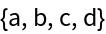Find a replacement path using universally quantified equations:

 In:=Out=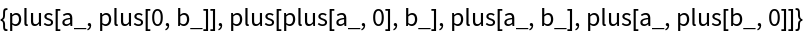In:=Out=In:=Out=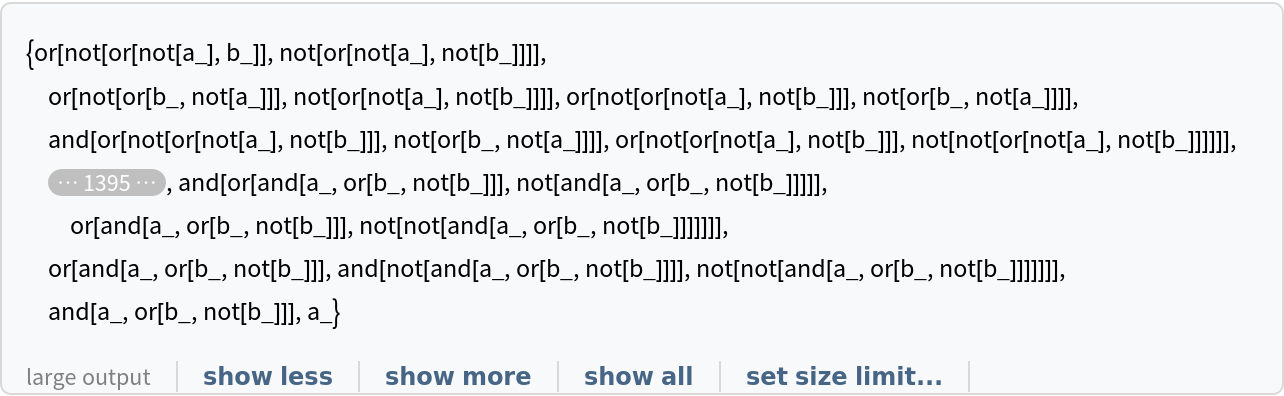In:=Out=In:=Out=### Scope (5)

Use names from AxiomaticTheory:

 In:=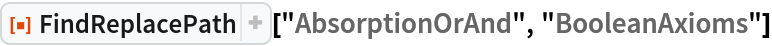Out=Use ProofObject to find a path:

 In:=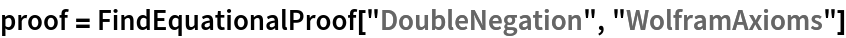Out=In:=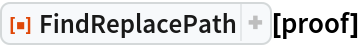Out=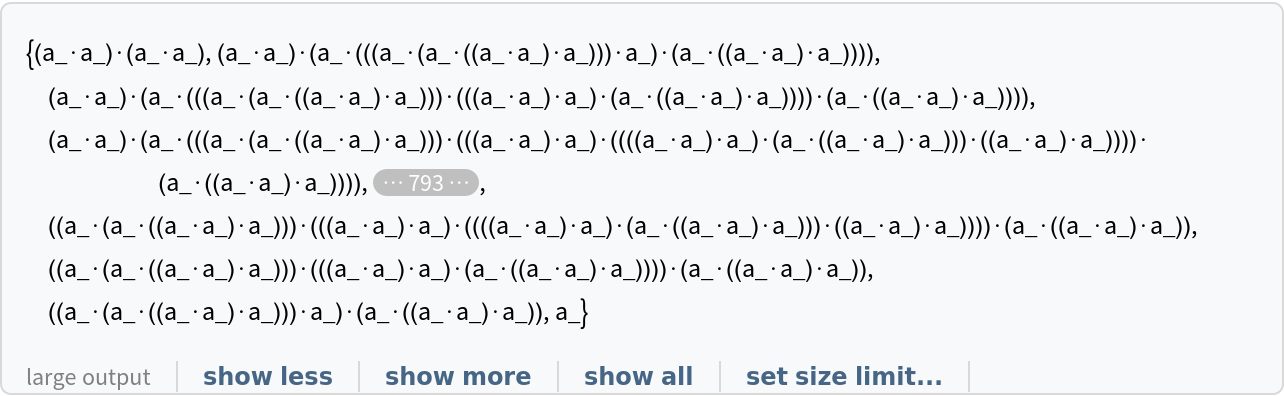Find a path in a string substitution system:

 In:=Out=In:=Out=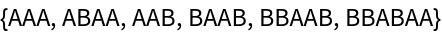Find a path in a Wolfram model system:

 In:=Out=In:=Out=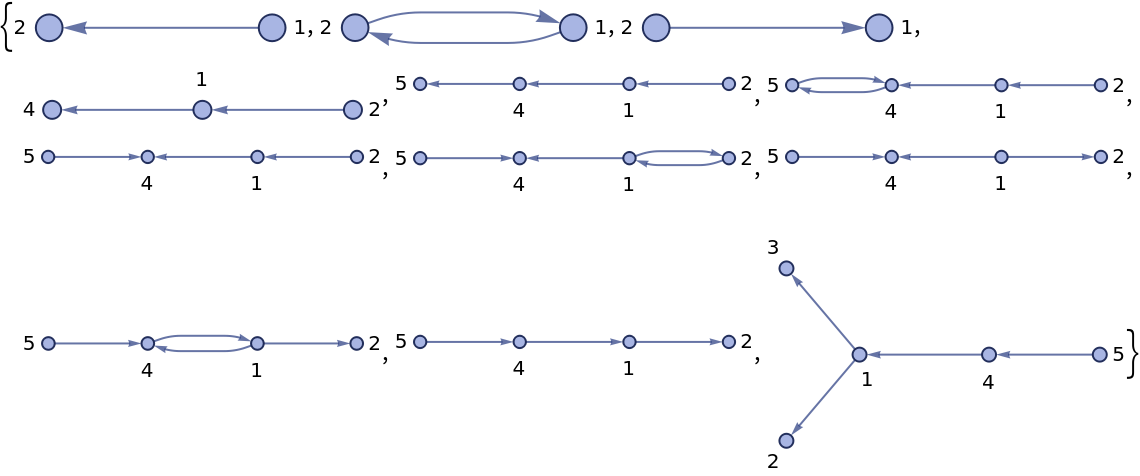Reproduce the found path using rewriting functions:

 In:=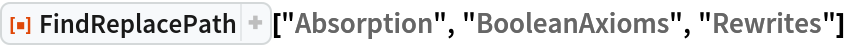Out=In:=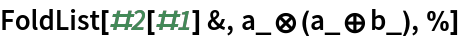Out=### Possible Issues (1)

Degenerate axiom systems capable of deriving a==b-type statements can sometimes fail:

 In:=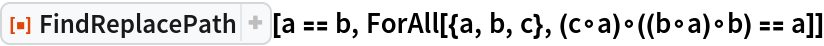Out=In:=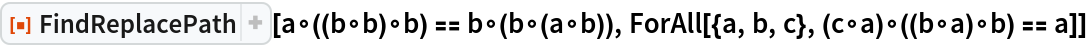Out=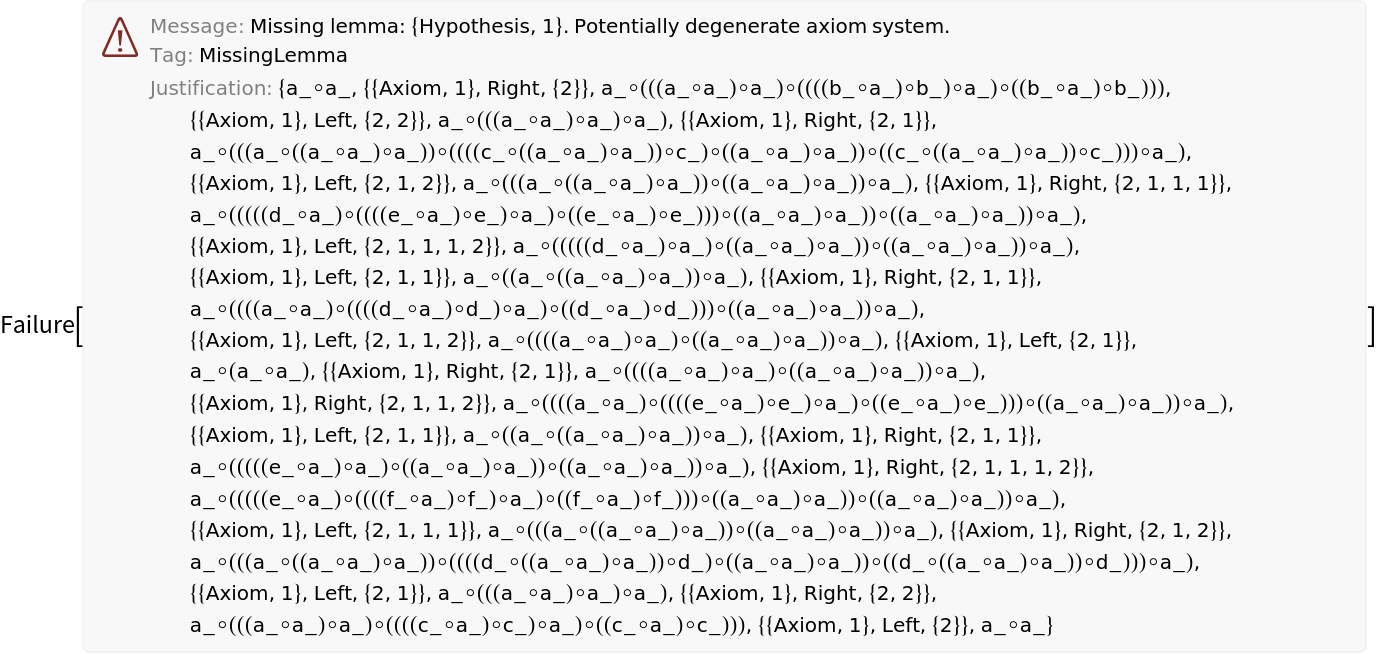### Neat Examples (2)

Test Boolean theorems:

 In:=Out=NKS example: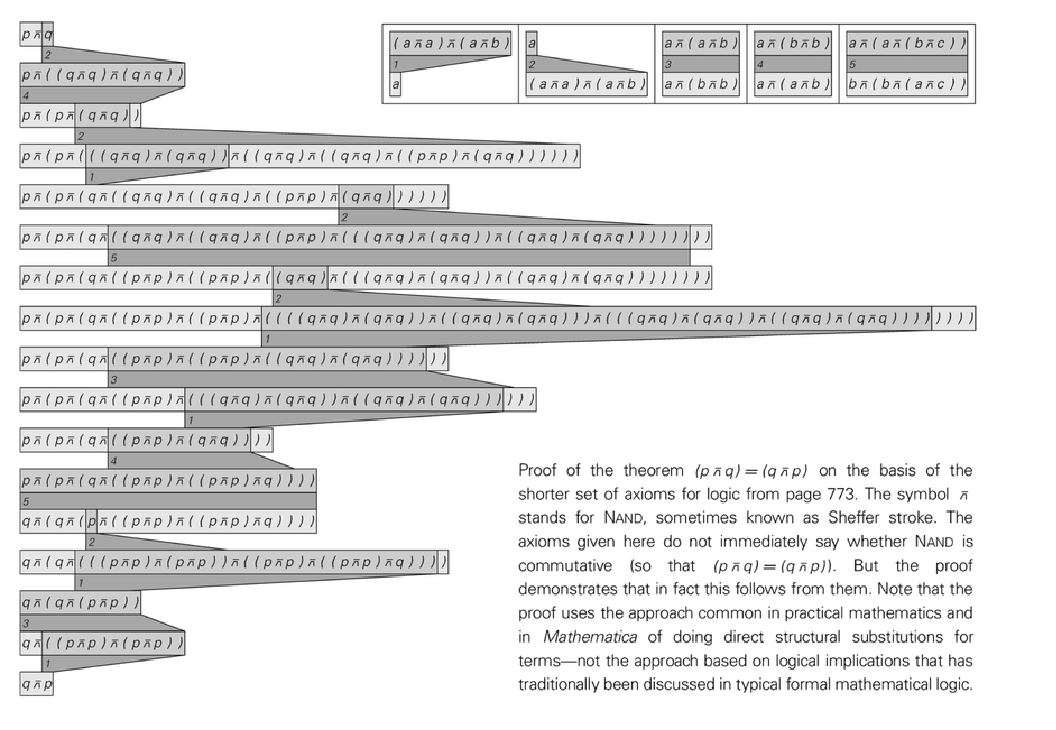In:=Out=In:=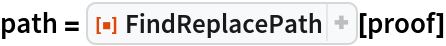Out=This result is two steps shorter than the NKS path, but it uses the reverse of one axiom:

 In:=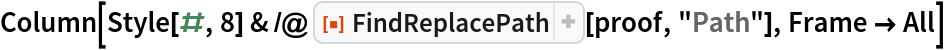Out=## Version History

• 1.0.0 – 07 February 2022